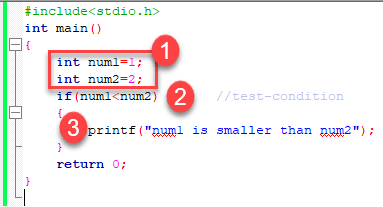# Conditional Statements in C | C Programming

Conditional Statements in C programming make decisions based on the given conditions in the command. They are executed in order or in a sequence when there is no condition around the statements. Thus, they are ‘decision making’ statements in C.

We can write conditional statements in C in these two ways:

1. If statement 2. If-else statement They are branching statements as they make a decision depending on the condition.

## If Statement

If statement is one of the powerful conditional statements. They are responsible for modifying the flow of execution of a program. And, the condition is evaluated followed by which any statement inside the body of If is excecuted. Syntax: The condition is given a true or false value. True – non-zero value False – zero value

```if (condition)
instruction; ```
##### Example: If construct to check if 2 numbers are equalThe numbers idicate the sequence in which the statements are excecuted.
##### Output:
`num1 is smaller than num2`

### Relational Operators

C has six relational operators. They can be used to formulate a Boolean expression for decision making and testing conditions. This returns true or false :

• < less than
• <= less than or equal to
• > greater than
• >= greater than or equal to
• == equal to
• != not equal to

The equal test (==) is different from the assignment operator (=).

#### Example:

```int x = 41;
x =x+ 1;
if (x == 42) {
printf("You succeed!");}
```
##### Output :
` You succeed `

## If-Else statement: extended if

Syntax:

```if (test-expression)
{
True block of statements
}
Else
{
False block of statements
}
Statements;```

#### Example:

##### Output:
`The value is greater than 10`

## Nested If-Else-if statements

When multipath decisions are needed to be taken, we use nested if else if.

Syntax:

```if (test - expression 1) {
statement1;
} else if (test - expression 2) {
Statement2;
} else if (test - expression 3) {
Statement3;
} else if (test - expression n) {
Statement n;
} else {
default;
}
Statement x;```

#### Example: Program to print the grade depending on scored marks.

##### Output:
`First class`

We will be happy to hear your thoughtsHello!👋